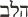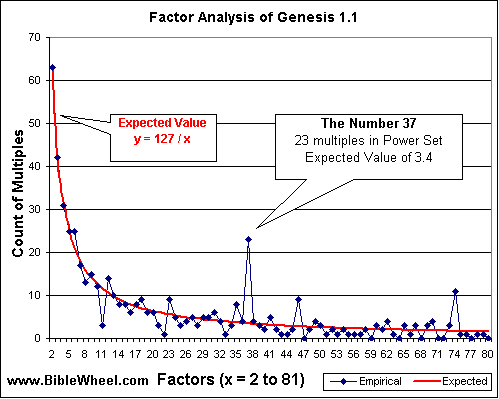Historical Archive of the Bible Wheel Site

The Bible Wheel had been debunked by its author.
Read all about it: Debunking Myself: What A Long Strange Trip It's Been

Recent Blog ArticlesIn the beginning God created the heaven and the earth.

Genesis 1.1

The centrality of the Prime Number 37 in the structure of Scripture can not be overstated. It begins in with the Creation Holograph:

Sum of Genesis 1.1 = 2701 = 37 x 73 = Sum(73)

The Number 37 is geometrically integrated with the Number 73 - it is the Hexagonal heart of 73 as Star, so 37 / 73 is a Hexagon / Star pair. The Number 37 is also the first number greater than unity that can be represented as both a Centered Hexagon and a Star:Such numbers are extremely rare. Here is a list of the first twelve numbers that are both Centered Hexagons and Stars:

1, 37, 1261, 42841, 1455337, 49438621, 1679457781, 57052125937, 1938092824081, 65838103892821, 2236557439531837, 75977114840189641

This sequence (and many others) can be viewed in the On-Line Encyclopedia of Integer Sequences (entry A006062).

As can be seen from the list, there are only twelve such numbers less than 7.5 x 1016, which is less than one in ten thousand trillion. The Number 37 is the only such number less than a thousand, and this is the Number found in the very heart of Genesis 1.1.

These Hexagon / Star pairs are deeply integrated with the fundamental words used in Scripture. Most obviously significant is the palindromic pair of primes (37 / 73) which are the Ordinal and Standard values of the fundamental Hebrew word Wisdom, by Which God created the world (Cf. Jeremiah 10.12):

 WisdomHokmah
37 = 8 + 11 + 13 + 5 (Ordinal Value)73 = 8 + 20 + 40 + 5 (Standard Value)

Note that the product of this gemometrically integrated pair of palindromic primes yields Genesis 1.1.

Exactly the same pattern - based now on the Hexagon/Star pair 19/37 - is found in the Hebrew word for The Heart:

 The HeartHaLev
19 = 5 + 12 + 2 (Ordinal Value)37 = 5 + 30 + 2 (Standard Value)

The product of these numbers also appear prominently in Genesis 1.1:

 and the earthV'eth HaAretz = 703 = 19 x 37 = Sum(37)

Thus, Genesis 1.1 can be written in two different ways in terms of Triangular Numbers:

Genesis 1.1 = Sum(73) = Sum(37) + 3 x Sum(36)

Note that the separation into the sum of Triangles is implicit within the grammatical structure of the verse!

The Number 37 also appears with exceedingly high frequency in the power set of Genesis 1.1. A power set is defined as the "set of all subsets of a set." So the power set of Genesis 1.1 contains every possible comination of its seven words. This yields 127 = 27 - 1 elements (since we are ignoring the empty set that is counted in the cardinality of the power set). The result is astounding. Below is the graph of the expected value, which can be calculated exactly, and the actual values generated from Genesis 1.1. The graph displays multiples less than 83 (after which the count is near zero anyway).Note the point corresponding to the Number 37. The numbers immediately surrounding it - 36 and 38 - coincide precisely with the expected value of about 3.4 multiples whereas the power set contains 23 combinations that are multiples of 37.

This phenomenon was first brought to my attention by Vernon Jenkins, in his detailed analysis of Genesis 1.1 called Inner Conviction. In the true scientific spirit, I reproduced his analysis to verify it and found we came to exactly the same conclusion. This was, of course, inevitable (sans error) since we are dealing with the objective reality of the text of the Holy Bible.